# Red and white

Simona picked 63 tulips in the garden and tied bicolor bouquets for her girlfriends. The tulips were only red and white. She put as many tulips in each bouquet, three of which were always red. How much could Simon tear off white tulips? Write all the options.

Result

b1 =  4
b2 =  6
b3 =  18

#### Solution:Leave us a comment of this math problem and its solution (i.e. if it is still somewhat unclear...):Be the first to comment!#### Following knowledge from mathematics are needed to solve this word math problem:

Do you solve Diofant problems and looking for a calculator of Diofant integer equations?

## Next similar math problems:

1. Toy carsPavel has a collection of toy cars. He wanted to regroup them. But in the division of three, four, six, and eight, he was always one left. Only when he formed groups of seven, he divided everyone. How many toy cars have in the collection?
2. Theorem proveWe want to prove the sentence: If the natural number n is divisible by six, then n is divisible by three. From what assumption we started?In six baskets, the seller has fruit. In individual baskets, there are only apples or just pears with the following number of fruits: 5,6,12,14,23 and 29. "If I sell this basket," the salesman thinks, "then I will have just as many apples as a pear." Which
4. Sheep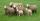Shepherd tending the sheep. Tourists asked him how much they have. The shepherd said, "there are fewer than 500. If I them lined up in 4-row 3 remain. If in 5-row 4 remain. If in 6-row 5 remain. But I can form 7-row." How many sheep have herdsman?
5. Nuts, girl and boys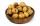Milena collected fallen nuts and called a bunch of boys let them share. She took a condition: the first boy takes one nut and tenth of the rest, the second takes 2 nuts and tenth new rest, the third takes 3 nuts and tenth new rest and so on. Thus managed.
6. Repair company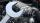The company repairs cars. The first day repair half of the contract second day, the half of the rest and third day 8 residue cars. How many total cars company repaired?
7. RemainderA is an arbitrary integer that gives remainder 1 in the division with 6. B is an arbitrary integer that gives remainder 2 the division by. What makes remainder in division by 3 product of numbers A x B ?
8. DivisorsThe sum of all divisors unknown odd number is 2112. Determine sum of all divisors of number which is twice of unknown numbers.
9. Balls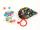Michal said to Martin: give me one ball and I'll have twice as you. Martin said: give me 4 and we will have equally. How many balls each have?
10. CandiesIf Alena give Lenka 3 candy will still have 1 more candy. If Lenka give Alena 1 candy Alena will hame twice more than Lenka. How many candies have each of them?
11. The diceWhat is the probability of events that if we throw a dice is rolled less than 6?
12. AntennasIf you give me two antennas will be same. If you give me again your two antenna I have a 5× so many than you. How many antennas have both mans?
13. Unknown numberUnknown number is divisible by exactly three different primes. When we compare these primes in ascending order, the following applies: • Difference first and second prime number is half the difference between the third and second prime numbers. • The produ
14. Three-digitHow many three-digit natural numbers do not have the number 7?
15. Seamstress 2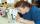Seamstress bought two kinds of textile in whole meters. One at 50 SKK and the second 70 SKK per meter. How many meter bought from this two textiles when paid totally 1540 SKK?
16. Numbers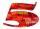Write smallest three-digit number, which in division 5 and 7 gives the rest 2.
17. Cows and calves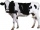There are 168 cows and calves in the cowshed. Cows are in nine stalls and calves in four stalls. Same count cows are in every cow stall and three more in each calf stall than in a cow stall. What is the capacity of the stalls for cows and what for calves?